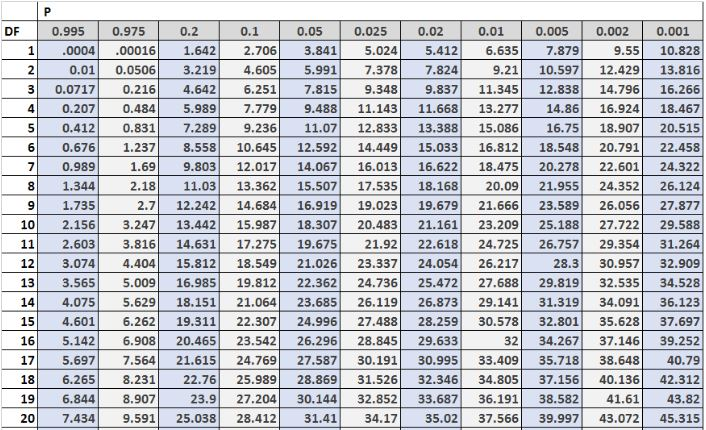# Implementation of Chi-Square in Research Project

There are research projects that require Implementation of Chi-Square in Research Project work, this article will walk you through on how to use Chi-Square in your research project.

First thing first, let define Chi-Square, Chi-Square is a statistical tool used to test the relationship between categorical variables.

The chi-square test is a statistical test that compares observed ( fo) and expected (fe) outcomes. The goal of this test is to figure out whether a disparity between observed and expected data is due to chance or a relationship between the variables you are looking at. As a result, using a chi-square test to better understand and interpret the relationship between our two category variables is an ideal choice.

The calculation of the Chi-Square statistic is quite straight-forward and intuitive:

where fo = the observed frequency (the observed counts in the cells)
and fe = the expected frequency if NO relationship existed between the variables

##### Implementation of Chi-Square in Research Project

For the purpose of this tutorial, we are going to adopt a sample question from Vector Academy, the table below is the Observed Values Table. The purpose of implementing Chi-Square is to if there is a relationship between Qualification Status and Marital Status. Qualification Status variable are highlighted in Red color while Marital Status variables highlighted in Blue.

#### Observed Values Table (fo)

We are going to set the hypotheses as follow:

Null Hypothesis Ho: There is no relationship between Marital Status and Qualification Status

Alternate Hypothesis H1: There is significant relationship between Marital Status and Qualification Status

Significance Level = 5% or 0.05

#### Expected Values Table (fe)

Expected values for Middle School and Never Married is calculated by multiplying total column value for Middle School and total row value for Never Married and divided by grand total of 300. Same procedures applies to the rest of the cells.

##### Calculation of Chi-Square X2 Table

The Chi-Square value of 23.57 is also known as Calculated Chi-Square.

The next step is to find the Tabulated Chi-Square value, firstly, we need to determine Degree of Freedom, it is calculated as follow:

DF = No of column – 1 X No of row – 1 (from the Observed Table)

Our Observed Table above has 5 columns and 4 rows.

therefore, DF = 5-1 X 4-1 = 12

##### How to find Tabulated Chi-Square

With the level of Significant Value given above as 5% or 0.05, we are going to trace both values of DF and Significance Level, this give us 21.026 being the value of Tabulated Chi-Square

#### Implementation of Chi-Square Analysis

Significance Level = 5% or 0.05

Calculated Chi-Square =23.57

Tabulated Chi-Square =21.03

In Chi-Square, if Calculated Chi-Square value is greater than Tabulated Chi-Square value, then Null Hypothesis (Ho) will be rejected while Alternate Hypothesis (H1) will be accepted.

#### Conclusion

There is significant relationship between Marital Status and Qualification Status

That how to Implement Chi-Square in Research Project

Recommended:

Student Guide: Final Year Project Report

How to Use Paraphrasing in Research Project Report

Scroll to Top